• 1
•
•
•
•
•
•
•
1
Share

# ELECTRIC POTENTIAL AND POTENTIAL DIFFERENCES

### OVERVIEW

Electric potential is a very important concept of electrodynamics. It is because the flow of electric charge is due to differences in electric potential. And charge motion is very important for study of current electricity. Without it we can’t think for electric current. So it is very important for us to understand the basic concepts of electric potential and electric potential difference and know what it is?, how it is create? And how how it tends to electron(electric charge) to flow? In this article we will talk about these in detail.

## ELECTRIC POTENTIAL

So what is electric potential? If we define it in simple words then we can say that it is nothing but the degree of electrification of the body. Because Electric potential is directly proportional to the charge. More charge more electric potential.Electric potential in the vicinity of two opposite point charges. Image source : Wikipedia.org

Electric potential of any body tells us about that how much a body is electrified. It also give the direction of flow of electric charge between the two charged bodies when they were put in contact.

### DEFINITION

The definition that we have talked above is that the degree of electrification is electric potential. It is quite simple. But in this definition, Can you tell me that how can we measure the electrification or how can we conclude that how much a body is electrified. If thought for it then we will find that it is somehow complex to explain it.

So we have to give a precise and formal definition of electric potential. The formal definition is –

The electrostatic potential at any point in the region of electric field is equal to the amount of work done in bringing the unit positive charge from a reference point to a specific point, against the electrostatic force with negligible acceleration.Typically, the reference point is the Earth or a point at infinity, mathematically, electrostatic potential is given as –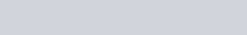According to the formula, we saw that work done by an external force in bringing a unit positive charge from a reference point to a specific point in electric field is equal to the electric potential (V) at that point.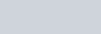Here value of electric potential is negative because work done by external force to bringing unit positive charge from infinity to a point in electric field is against the electrostatic force. This work done per unit charge is stored in electrical bodies as electric potential.
It’s SI unit is volt(V) and it’s dimensional formula is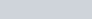Electric potential at a point is said to be one volt, when one joule of work is done in bringing one coulomb of positive charge from infinity to a specific point in electric field, against the electrostatic force, without any acceleration.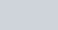## POTENTIAL DIFFERENCE

So far we have talked about electric potential, now we will talk about electric potential difference. We just saw that electric potential is amount of work done in bringing a unit positive charge from infinity to a specific point in electric field, against electrostatic force without any acceleration. But what, this definition can also apply for potential difference?

No, we can’t apply this definition for the electric potential difference. In the definition of electric potential, positive charge was bringing from infinity to a specific point. But in the definition of electric potential difference positive charge are taken from one point to another point in same electric field.

### DEFINITION

Electric potential difference formally can be defined as –

Electric potential difference between the two points P and Q is equal to the work done by the external force in moving a unit positive charge against the electrostatic force from point Q to P along any path taken between these two points.

The SI unit of electric potential difference is volts (V).

Following figure show that work done on a test charge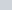by the electrostatic force due to any given charge configuration depends only on the initial position point Q and final position point P. Work done is independent of the path taken in going from point B to point P.

If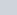and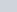are the electric potential at point Q and point P respectively, then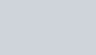The dimensional formula of electric potential difference is-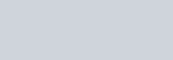And the SI unit electric potential difference is volt.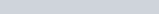Thus, electrostatic potential difference between any two points P and Q in an electrostatic field is said to be one volt, when one joule of work is done by external force in moving a positive charge of one coulomb from one point to the other point against the electrostatic force of the field without any acceleration.

### FREQUENTLY ASKED QUESTIONS ON ELECTROSTATIC POTENTIAL AND POTENTIAL DIFFERENCES

1). What is meant by electric potential?

Ans- The simple meaning of electric potential is that the amount of work done in bringing a unit positive test charge from infinity to a specific point in electric field E against the electrostatic force without any acceleration.

2). What does a positive Electric potential means?

Ans- when we say positive electric potential then our means that positive electric charge at that point will have have higher electric potential energy as compared to its reference point. Or negative electric charge at that point will have lower potential energy as compared to its reference point.

3). How can electric potential used?

Ans- Electric potential can be used to charge a body of low electric potential. When a low electric potential body keep in contact with the body of high electric potential then there’s create a difference in potential called potential difference.

After creating potential difference, electrons started moving from the body of high potential to the body of low potential untill and unless they attain equal potential. This motion of electric charge(electron) also creates a electric current.

3). What is electric potential difference in simple words?

Ans- In simple words potential difference is nothing but it is only difference in electric potential.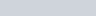4). Why electric potential is scalar?

Ans-  Electric potential is scalar because it is a derived quantity. It is derived from Potential energy. If we divide potential energy of a test charge with its charge at every location of space, we got a quantity called electric potential.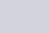5). Can electric potential be zero.

Ans- yes, electric potential can be zero, if the point is placed at infinity from the source field then for that point Electric potential will be zero. When you come towards the positive charge from infinity then the electric potential will gradually increase and if you go away from it, then it gradually decreases.

6). What will be the nature of electric potential at a place where the electric field is zero?

Ans- If electric field is zero, then if we bring a unit positive charge from infinity to a point in the region of zero electric field. Then no work is done by the external force because there is no any opposing force due to zero electric field. If work is zero then electric potential will be zero.